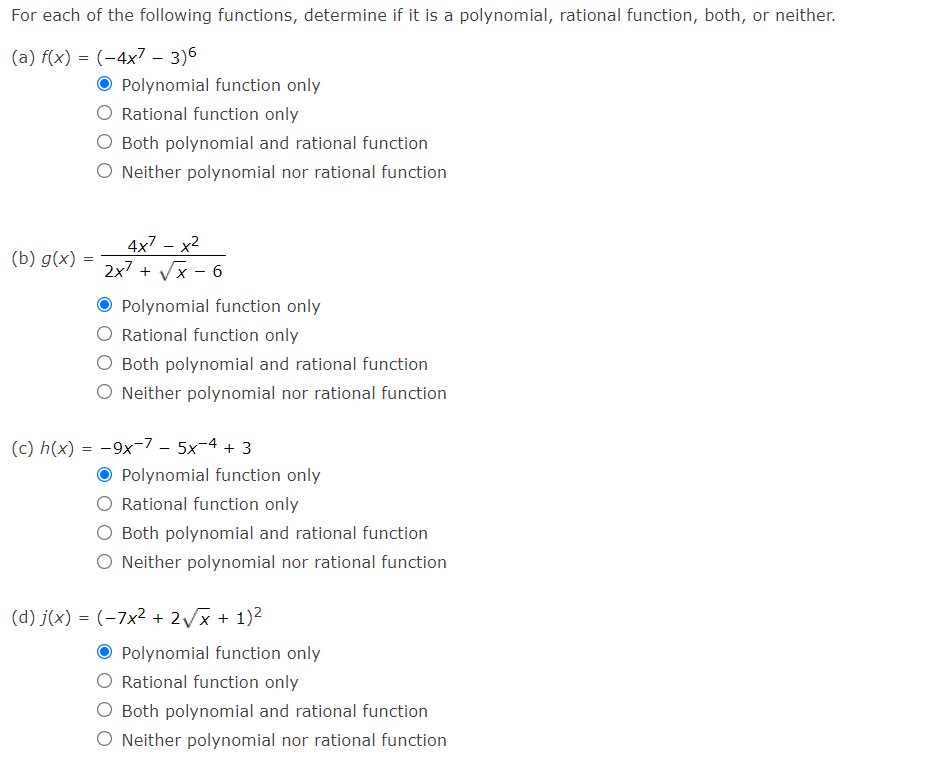Home / Expert Answers / Calculus / for-each-of-the-following-functions-determine-if-it-is-a-polynomial-rational-function-both-or-pa649

# (Solved): For each of the following functions, determine if it is a polynomial, rational function, both, or ...For each of the following functions, determine if it is a polynomial, rational function, both, or neither. (a) $$f(x)=\left(-4 x^{7}-3\right)^{6}$$ Polynomial function only Rational function only Both polynomial and rational function Neither polynomial nor rational function (b) $$g(x)=\frac{4 x^{7}-x^{2}}{2 x^{7}+\sqrt{x}-6}$$ Polynomial function only Rational function only Both polynomial and rational function Neither polynomial nor rational function (c) $$h(x)=-9 x^{-7}-5 x^{-4}+3$$ Polynomial function only Rational function only Both polynomial and rational function Neither polynomial nor rational function (d) $$j(x)=\left(-7 x^{2}+2 \sqrt{x}+1\right)^{2}$$ Polynomial function only Rational function only Both polynomial and rational function Neither polynomial nor rational function

We have an Answer from Expert